## ERNIE - Echelons of Residential Neighbourhoods (IE)

### National Centre for Geocomputation, National University of Ireland Maynooth

This function takes a 'raw' data frame from the Irish Census, and returns a data frame of derived variables, designed to be a close match to those used for the UK OAC classification of Uk Census Output Areas.

CreateVariables <- function(CensusData,na.rm=TRUE) {
attach(CensusData)
Age0_4    <- 100 * ( T1_1AGE0T + T1_1AGE1T + T1_1AGE2T + T1_1AGE3T + T1_1AGE4T ) /
T1_1AGETT
Age5_14   <- 100 * ( T1_1AGE5T + T1_1AGE6T + T1_1AGE7T + T1_1AGE8T + T1_1AGE9T +
T1_1AGE10T + T1_1AGE11T + T1_1AGE12T + T1_1AGE13T + T1_1AGE14T) /
T1_1AGETT
Age25_44  <- 100 * ( T1_1AGE25_29T + T1_1AGE30_34T + T1_1AGE35_39T + T1_1AGE40_44T ) /
T1_1AGETT
Age45_64  <- 100 *( T1_1AGE45_49T + T1_1AGE50_54T + T1_1AGE55_59T + T1_1AGE60_64T ) / T1_1AGETT
Age65over <- 100 *( T1_1AGE65_69T + T1_1AGE70_74T + T1_1AGE75_79T + T1_1AGE80_84T + T1_1AGEGE_85T ) / T1_1AGETT

EU_National           <- 100 * (T2_1UKN + T2_1PLN + T2_1LTN + T2_1EUN) / T2_1TN
ROW_National          <- 100 * (T2_1RWN) / T2_1TN
Born_outside_Ireland  <- 100 * (T2_1TBP - T2_1IEBP) / T2_1TBP

Separated            <- 100 * (T1_2SEPT + T1_2DIVT) / T1_2T
SinglePerson         <- 100 * (T5_2_1PP - T4_5RP  ) / T5_2_TP
Pensioner            <- 100 * T4_5RP / T4_5TP
LoneParent           <- 100 * (T4_3FTLF + T4_3FTLM) / T4_5TF
DINK                 <- 100 * T4_5PFF / T4_5TF
NonDependentKids     <- 100 * T4_4AGE_GE20F / T4_4TF

RentPublic         <- 100 * T6_3_RLAH / T6_3_TH
RentPrivate        <- 100 * T6_3_RPLH / T6_3_TH
#Terraced           <-
#Detached           <-
Flats              <- 100 * T6_1_FA_H / T6_1_TH
NoCenHeat          <- 100 * T6_5_NCH / T6_5_T
RoomsHH            <- (T6_4_1RH + 2*T6_4_2RH + 3*T6_4_3RH + 4*T6_4_4RH + 5*T6_4_5RH + 6*T6_4_6RH + 7*T6_4_7RH + 8*T6_4_GE8RH) / T6_4_TH
PeopleRoom         <- T1_1AGETT / (T6_4_1RH + 2*T6_4_2RH + 3*T6_4_3RH + 4*T6_4_4RH + 5*T6_4_5RH + 6*T6_4_6RH + 7*T6_4_7RH + 8*T6_4_GE8RH)
SepticTank         <- 100 * T6_7_IST / T6_7_T

HEQual              <-  100 * ((T10_4_ODNDT + T10_4_HDPQT + T10_4_PDT + T10_4_DT) / T10_4_TT) # educ to degree or higher
Employed            <-  100 * T8_1_WT / T8_1_TT
TwoCars             <-  100 * (T15_1_2C + T15_1_3C + T15_1_GE4C) / (T15_1_NC + T15_1_1C + T15_1_2C + T15_1_3C + T15_1_GE4C)
JTWPublic           <-  100 * (T11_1_BU + T11_1_TDL) / T11_1_T
HomeWork            <-  100 * T9_2_PH / T9_2_PT
LLTI                <-  100 * (T12_3BT + T12_3VBT) / T12_3TT
UnpaidCare          <-  100 * (T12_2TM + T12_2TF )/ (T1_1AGETT)

Students           <- 100 * T8_1_ST / T8_1_TT
Unemployed         <- 100 * T8_1_ULGUPJT / T8_1_TT
# PartTime           <- 100 *
EconInactFam       <- 100 * T8_1_LAHFT / T8_1_TT
Agric              <- 100 * (T14_1_AFFM + T14_1_AFFF) / (T14_1_TM + T14_1_TF)
Construction       <- 100 * (T14_1_BCM  + T14_1_BCF ) / (T14_1_TM + T14_1_TF)
Manufacturing      <- 100 * (T14_1_MIM  + T14_1_MIF ) / (T14_1_TM + T14_1_TF)
Commerce           <- 100 * (T14_1_CTM  + T14_1_CTF ) / (T14_1_TM + T14_1_TF)
Transport          <- 100 * (T14_1_TCM  + T14_1_TCF ) / (T14_1_TM + T14_1_TF)
Public             <- 100 * (T14_1_PAM  + T14_1_PAF ) / (T14_1_TM + T14_1_TF)
Professional       <- 100 * (T14_1_PSM  + T14_1_PSF ) / (T14_1_TM + T14_1_TF)

### MISC
Broadband          <- 100 * T15_3_B / (T15_3_B + T15_3_OTH)            # Internet connected HH with Broadband
Internet           <- 100 * (T15_3_B + T15_3_OTH) / T15_3_T            # Households with Internet

detach(CensusData)
### Bringing it all together

Place <- data.frame(CensusData[,1],stringsAsFactors=FALSE)
colnames(Place) <- 'GEOGID'
Demographic <- data.frame(Age0_4, Age5_14, Age25_44, Age45_64, Age65over, EU_National, ROW_National, Born_outside_Ireland)
HouseholdComposition <- data.frame(Separated, SinglePerson, Pensioner, LoneParent, DINK, NonDependentKids)
Housing <- data.frame(RentPublic, RentPrivate, Flats, NoCenHeat, RoomsHH, PeopleRoom, SepticTank)
SocioEconomic <- data.frame(HEQual, Employed, TwoCars, JTWPublic, HomeWork, LLTI, UnpaidCare)
Employment <- data.frame(Students, Unemployed, EconInactFam,Agric,Construction,Manufacturing,Commerce,Transport,Public,Professional)

DerivedData <- data.frame(Place,Demographic,HouseholdComposition,Housing,SocioEconomic,Employment,Misc)
if (na.rm) DerivedData[which(is.na(DerivedData),arr.ind=T)] <- 0                            # there are a few NAs - not when I do it? - CB

DerivedData
}


### Obtaining the data

These were incorporated by Chris Brunsdon into the R ie11census package who downloaded all SAPS data via the link http://www.cso.ie/en/media/csoie/census/documents/saps2011files/Complete_Set_Of_SAPS_2011.zip on the page http://www.cso.ie/en/census/census2011smallareapopulationstatisticssaps/. This information is supplied to facilitate reproducibility.

Firstly read the data - use the ie11census package to do this

library(ie11census)

# SA - small areas This gets the raw data



Next compute the derived variables

# This transforms it



This provides a working data set. The next stage is to use k-means cluster analysis  to derive the classification.

# The Area Classification Code

This creates a national Small Area Classification (ERNIE - Echelons of Residential Neighbourhoods - IE) - see  for an outline of the classification methodology. The first step is a principal components analysis:

PCA <- princomp(SAVars[, -1], cor = T, scores = T)
PCA$sdev^2/sum(PCA$sdev^2)

##    Comp.1    Comp.2    Comp.3    Comp.4    Comp.5    Comp.6    Comp.7
## 0.2444728 0.1405325 0.0881169 0.0735823 0.0446917 0.0360247 0.0286841
##    Comp.8    Comp.9   Comp.10   Comp.11   Comp.12   Comp.13   Comp.14
## 0.0273957 0.0246839 0.0238798 0.0210039 0.0207057 0.0191204 0.0186719
##   Comp.15   Comp.16   Comp.17   Comp.18   Comp.19   Comp.20   Comp.21
## 0.0168411 0.0163330 0.0150647 0.0138634 0.0135201 0.0125949 0.0115549
##   Comp.22   Comp.23   Comp.24   Comp.25   Comp.26   Comp.27   Comp.28
## 0.0108989 0.0102042 0.0092108 0.0080217 0.0067576 0.0063148 0.0057492
##   Comp.29   Comp.30   Comp.31   Comp.32   Comp.33   Comp.34   Comp.35
## 0.0052284 0.0045675 0.0039899 0.0034871 0.0029046 0.0025246 0.0024934
##   Comp.36   Comp.37   Comp.38   Comp.39   Comp.40
## 0.0022130 0.0016964 0.0011062 0.0009463 0.0003471


The above lists the proportion of variance than each component explains. The next step is to investigate the cumulative amount of variance explained.

cumsum(PCA$sdev^2/sum(PCA$sdev^2))

##  Comp.1  Comp.2  Comp.3  Comp.4  Comp.5  Comp.6  Comp.7  Comp.8  Comp.9
##  0.2445  0.3850  0.4731  0.5467  0.5914  0.6274  0.6561  0.6835  0.7082
## Comp.10 Comp.11 Comp.12 Comp.13 Comp.14 Comp.15 Comp.16 Comp.17 Comp.18
##  0.7321  0.7531  0.7738  0.7929  0.8116  0.8284  0.8447  0.8598  0.8737
## Comp.19 Comp.20 Comp.21 Comp.22 Comp.23 Comp.24 Comp.25 Comp.26 Comp.27
##  0.8872  0.8998  0.9113  0.9222  0.9324  0.9417  0.9497  0.9564  0.9627
## Comp.28 Comp.29 Comp.30 Comp.31 Comp.32 Comp.33 Comp.34 Comp.35 Comp.36
##  0.9685  0.9737  0.9783  0.9823  0.9858  0.9887  0.9912  0.9937  0.9959
## Comp.37 Comp.38 Comp.39 Comp.40
##  0.9976  0.9987  0.9997  1.0000


Note that the first 14 components account for 81.2% of the variance in total. We will use these for a k-means cluster analysis. Note that n.pc in the code refers to the number of principal components considered.

set.seed(290162)  # Reproducible outcome
library(lubridate)
then <- now()  # Time this - it takes a while

smallest.clus <- wss <- rep(0, 100)

for (i in 1:100) {
clus <- kmeans(PCA$scores[, 1:n.pc], i, iter.max = 100, nstart = 20) wss[i] <- clus$tot.withinss
smallest.clus[i] <- min(clus$size) } # How long did the calculation take? elapsed <- now() - then  plot(1:100, wss[1:100], type = "h", main = "Cluster Scree Plot", xlab = "Number of Clusters", ylab = "Within Cluster Sum of Squares")A further issue is the size of the clusters. One problem frequently occuring with $$k$$-means cluster analysis is that very unusual cases tend to break of into small clusters - largely because they aim to minimise the within-cluster sum of squares, and small groups of similar but outlying observations contribute highly to this quantity unless they are assigned their own cluster. This sometimes means that increasing the number of clusters tends to create these very small 'outlier' clusters. A helpful diagnostic is to plot the smallest cluster size against the total number of clusters: plot(1:100, smallest.clus[1:100], type = "h", main = "Smallest Cluster Plot", xlab = "Number of Clusters", ylab = "Smallest Cluster Size")From this it seems that the 'very small cluster' phenomenon starts to occur when the number of clusters exceeds 18 - assuming a very small cluster has fewer than 15 small areas contained within itself. This took 11.94 mins to complete on a 3.5 GHz Intel Core i7 iMac. Here, the classification for 18 clusters is re-calculated - this quantity is stored in n.clus in the code below. This will be used as it provides a reasonable trade off between within cluster sum of squares and minimum cluster size. # set seed to get the same answer each time! set.seed(32767) SAclus <- kmeans(PCA$scores[, 1:n.pc], n.clus, iter.max = 100, nstart = 20)

SAclusters <- SAclus$cluster  ## Diagnostics The purpose of this section is to identify the characteristics of the clusters found above. Since the above computation applied the analysis to principal components it is helpful to characterise the clusters in terms of the original variables used in the PCA. To do this, firstly the $$z$$-scores of each variable for the cluster centroids are computed. If the initial variable is $$x$$, then the $$z$$-score is given by $z = \frac{x - \bar{x}}{s}$ where $$\bar{x}$$ is the arithmetic mean of the $$x$$ values in the data set, and $$s$$ is the sample standard deviation defined by $s = + \sqrt{\frac{\sum{(x - \bar{x})^2}}{n - 1}}$ this standardisation allows values of each variable to be compared on a consistent scale - the $$z$$-score in each case has a mean of zero and a standard deviation of one. Here, the $$x$$ values are actually cluster-wise mean values, whereas $$\bar{x}$$ and $$s$$ are computed for values for all Small Areas. This is useful for identifying which clusters have relatively high or low values of particular variables. The following code creates a set of $$z$$ scores for each cluster. # We need this for the 'ddply' function library(plyr) # Compute a data frame (one row per cluster) containing the means of each # variable in that cluster mean_by_cluster <- ddply(SAVars, .(SAclusters), numcolwise(mean))[, -1] # Compute the column-wise means for *all* observations mean_by_col <- apply(SAVars[, -1], 2, mean) # Compute the column-wise *sample* sd's for *all* observations sd_by_col <- apply(SAVars[, -1], 2, sd) # Create the z-scores via the 'scale' function z_scores <- scale(mean_by_cluster, center = mean_by_col, scale = sd_by_col)  The above code used the ddply function in the plyr package. The **ply function in this package is used to split the data frame according to a variable (here SAclusters) - it is an example of a split-apply-combine operation. The data frame is split into a number of sub-frames according to the value of SAClusters, then an operation is applied to each sub-frame (in this case computing a list of column means - the expression numcolwise(mean) takes a function mean and creates a new function, that applies mean to each numeric column in a data frame), and finally these are combined together to create a single data frame. These may now be explored visually. The heatmap function provides a visual representation of the z_scores variable. Essentially it provides a grid-based image of the values in the two dimensional array. However, noting that the column and row labels are both categorical, non-ordinal variables. Although he cluster numbers are integers, no significance is attached to their values - they merely serve as labels. This implies that both rows and columns may be permutated, without loss of generality. Doing this can sometimes aid the clarity of the visualisation. In this case, rows are re-ordered on the basis of applying a dendrogram ordering algorithm to the result of a hierarchical clustering algorithm, where the values along each row are treated as a vector. The clustering is carried out on the basis of Euclidean distance between the vectors using the complete linkage  approach, and the re-ordering is based on the reorder.dendrogram function provided in the R stats package provided in standard distributions of R. As noted, this re-ordering is applied to the rows, but following this, a re-ordering is also applied to the columns - this time treating the columns as vectors, and computing Euclidean distances between these. library(RColorBrewer) heatmap(t(z_scores), scale = 'none', col=brewer.pal(6,'BrBG'), breaks=c(-1e10,-2,-1,0,1,2,+1e10), xlab='Cluster Number', add.expr=abline(h=(0:40)+0.5,v=(0:n.clus)+0.5,col='white'))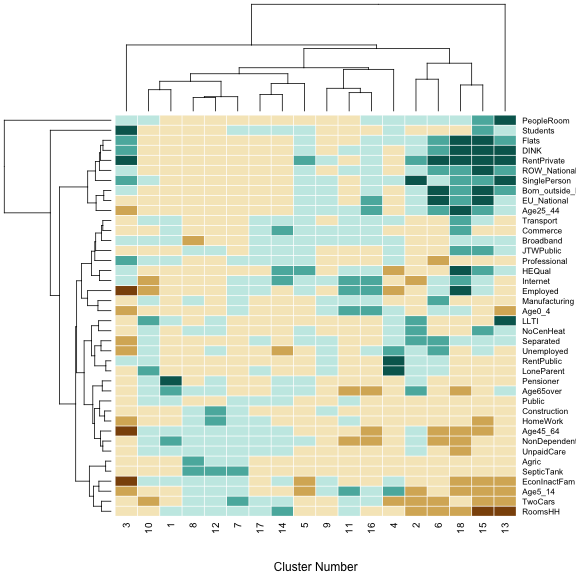Some further details are that the colouring of the pixels is based on the Brewer 'BrBG' palette - see eg http://colorbrewer2.org - with category cut-off points at -2, -1, 0, 1, and 2 - thin white lines are added to the grid so that the divisions between individual rows and columns can be seen. Finally, the dendrograms are actually drawn against the axes, so that hierarchical groups of the $$k$$-means clusters, and the of variables can be seen. The former is helpful in providing a hierarchical higher-level grouping of clusters, based on their similarities. It is also a useful detector for 'clusters of outliers' as described earlier. For example, the cluster dendrogram in the figure above suggests that cluster 13 is unusual (note that heirarchically, it is split away into a one element group before any other divisions are encountered), and a similar observation can be made for cluster 3. This dendrogram for the variable also suggests that the 'People per room' is unusual as it is quite distinct from all others in terms of its representation across the clusters. A final evaluation is to investigate the distribution of group sizes for this particular classification: barplot(sort(table(SAclusters)), las = 3)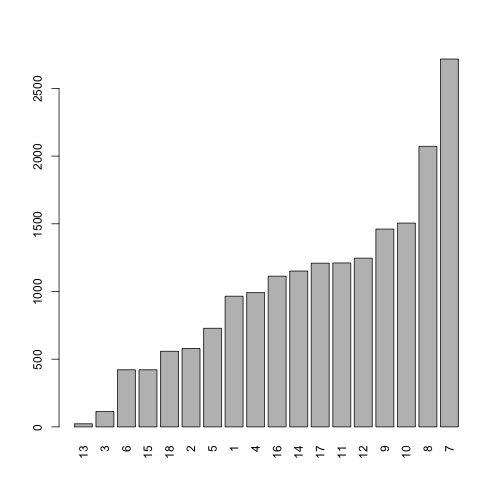Although there is a fairly even spread of sizes, there is quite a difference between the smallest (23) and largest (2717) - a dynamic range of around 100. ## Using PAM Instead of $$k$$-Means An alternative clustering technique is Kaufman and Rousseeuw's Partitioning Around Medoids (PAM) algorithm . A key difference between this and $$k$$-means is that the total absolute distances within clusters are minimised, rather than the sum of squared distances. The contribution to this sum from outliers is proportionally less, and so there is less tendency to form small clusters of outlying areas. However, it should be noted that a single run of this algorithm for every small area in Ireland takes notably longer than an equivalent run of $$k$$-means. # set seed to get the same answer each time! library(cluster) set.seed(32767) then2 <- now() PAMclus <- pam(x = PCA$scores[, 1:n.pc], k = n.clus, pamonce = 2)
elapsed2 <- now() - then2
PAMclusters <- PAMclus$clustering  This algorithm took 9.261 mins to run. Note that this was just to run a single classification, compared to the time stated earlier (11.94 mins) for 100 runs of $$k$$-means classificatuions. This isn't the fastest milk float in the west, unfortunately. However, the algorithm only needs to be run one time to obtain the classification, so now the diagnostic tests that were run on the $$k$$-means result can be run here. # Repeat z-score analysis with pam-based clusters # Compute a data frame (one row per cluster) containing the means of each # variable in that cluster mean_by_cluster2 <- ddply(SAVars, .(PAMclusters), numcolwise(mean))[, -1] # Create the z-scores via the 'scale' function z_scores2 <- scale(mean_by_cluster2, center = mean_by_col, scale = sd_by_col)  heatmap(t(z_scores2), scale = 'none', col=brewer.pal(7,'BrBG'), breaks=c(-1e10,-2,-1,-0.5,0.5,1,2,+1e10), xlab='Cluster Number', add.expr=abline(h=(0:40)+0.5,v=(0:n.clus)+0.5,col='white'))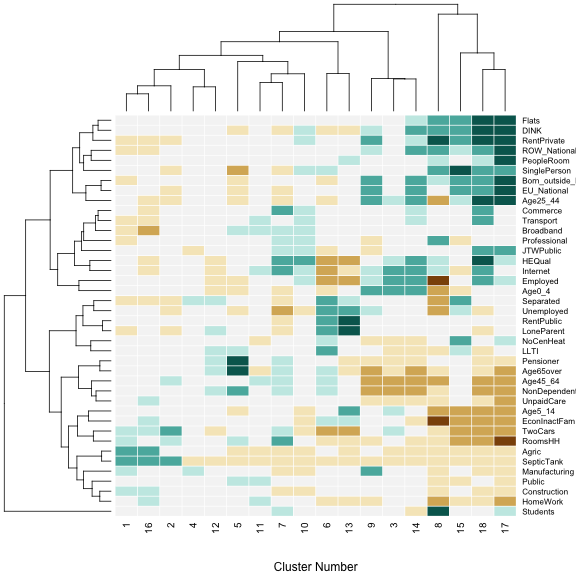barplot(sort(table(PAMclusters)), las = 3)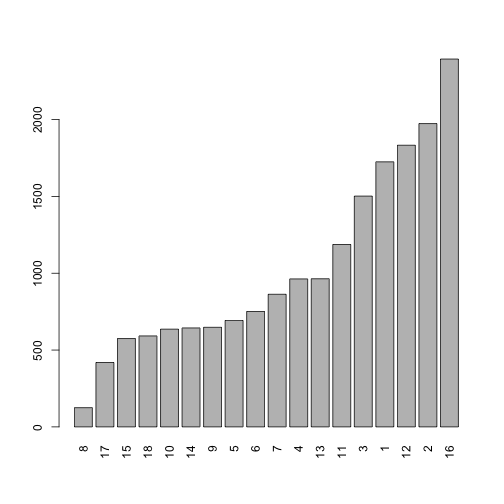This time, although there is still some spread of sizes, it is much less than with $$k$$-means, there is less contrast between the smallest (125) and largest (2394) - a dynamic range of around 20. library(MASS) mds.locs2 <- sammon(dist(z_scores2)) plot(mds.locs2$points, type = "n", xlab = "Dimension 1", ylab = "Dimension 2",
asp = 1)
text(mds.locs2$points, label = paste("Group", 1:18))### Mapping Diagnostics As well as investigating relationships between the input variables for the cluster analysis, it is illuminating to map the geographical distributions of distinct clusters. This is done below. However a single map for the whole of the Republic in a standard map projection is not helpful - the areal units used are Small Areas, and although fairly uniform in population, their physical sizes vary notably, so that on a map containing the entire Republic, urban Small Areas are too small to be visible. One strategy to address this problem is to use cartograms - for example using the algorith set out in . However, a complication is that such projections distort relatively familiar geographical shapes. Thus, for this exploration, instead of cartograms, Tufte's principle of small multiples  is invoked. For each group, small areas belonging to that group are shaded in six different map views - the five settlements in the Republic of Ireland classed as 'cities' - or more accurately have a name in the 'Settlement' OSI shape file ending in '… city and suburbs'. These correspond to the places Waterford, Galway, Limerick, Cork and Dublin. The sixth view is of the Republic of Ireland as a whole. 'Zooming in' to cities in this way shows detailed patterns around major centres of population, where Small Areas have very little area, and more detail is needed. The 'whole Republic' view is more helpful for clusters that are more rural in character. Note that the 'city' views are essentially a city and its suburbs. In each of these views, the areas outside the boundary for each city and suburbs is partially greyed out. This greying is translucent - allowing the areas within a given cluster but outside the boundary to be seen, but distinguishing these areas from thoses within the city and suburbs. The code used to create these maps is shown below, followed by 18 6-view maps, one for each of the groups. library(ie11census) library(GISTools) data(boundaries) clus.spdf <- polys.ie(SA.spdf, cbind(SAVars, PAMclusters)) # Create a set of city boundaries, chosen by 'city' appearing in the # settlement name invisible(Sys.setlocale("LC_ALL", "C")) # This stops R going into conniptions about 'invalid' characters (actually Irish accents) in the place names. city.spdf <- ST.spdf[grep("city", ST.spdf$SETTL_NAME), ]  # Find all the settlements with 'city' in their name

# Create an spdf containing an expanded bounded box with the input spdf
# around it
get.box <- function(q) {
bbx <- q@bbox
bbx.x <- bbx[1, c(1, 2, 2, 1, 1)]
bbx.y <- bbx[2, c(1, 1, 2, 2, 1)]
bbx.x <- 3 * (bbx.x - mean(bbx.x)) + mean(bbx.x)
bbx.y <- 3 * (bbx.y - mean(bbx.y)) + mean(bbx.y)
res <- readWKT(sprintf("MULTIPOLYGON (((%s )))", paste(sprintf("%f %f",
bbx.x, bbx.y), collapse = ", ")))
proj4string(res) <- proj4string(q)
return(res)
}

# Create same as above, but with a hole shaped like input spdf cut into it
get.holey.box <- function(q) gDifference(get.box(q), q)

# Make the 6-way small multiple map
make.map <- function(gp, colour, hdr) {
par(mfrow = c(2, 3), mar = c(1, 1, 3, 1))
for (i in 1:5) {
plot(city.spdf[i, ], col = NA, border = NA)
plot(gp, add = TRUE, col = colour, border = NA)
plot(get.holey.box(city.spdf[i, ]), add = TRUE, border = NA, col = rgb(0,
0, 0, 0.1))
title(city.spdf[i, ]$SETTL_NAME, cex.main = 1.5) box(which = "figure") } plot(PR.spdf) plot(gp, add = TRUE, col = colour, border = NA, lwd = 0.5) plot(get.holey.box(PR.spdf), col = "lightblue", add = TRUE, border = NA) title(paste0("All of RoI: ", hdr), cex.main = 1.5) box(which = "figure") cat(" \n\n\n") par(oldpar) } # Sample of random shades for the ERNIE groups - a random selection with no # sequential repeats sample.norun <- function(ls, n) { smp <- sample(ls, 1) if (n == 1) return(smp) last.one <- smp for (i in 2:n) { this.one <- sample(setdiff(ls, last.one), 1) smp <- c(smp, this.one) last.one <- this.one } return(smp) } # Choose the colours colchoice <- sample.norun(c("darkblue", "darkgreen", "darkred", "darkorange", "darkgoldenrod"), 18) # Create the multimaps for (i in 1:n.clus) { cat(sprintf("Group %d\n", i)) clusi.spdf <- clus.spdf[clus.spdf$PAMclusters == i, ]
make.map(clusi.spdf, colchoice[i], paste("Group", i))
}

## Group 1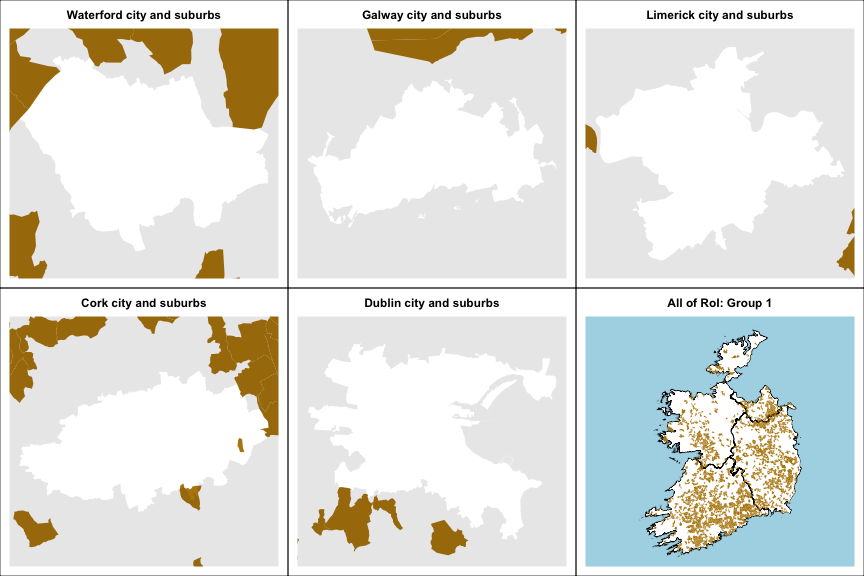##
##
##
## Group 2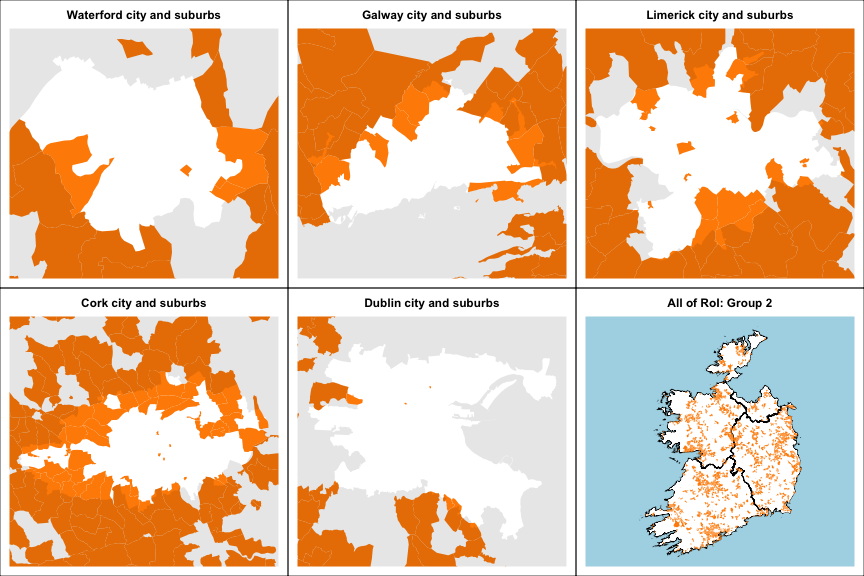##
##
##
## Group 3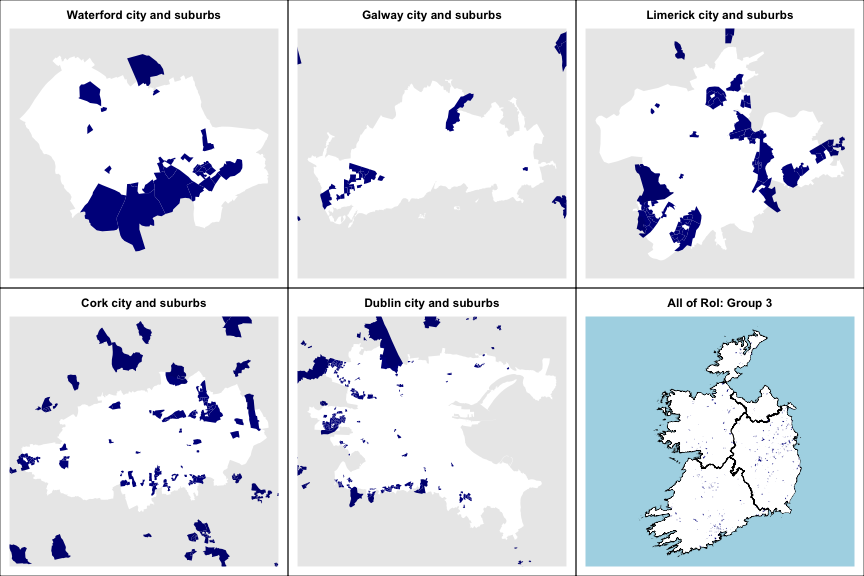##
##
##
## Group 4